Algorithmic Data Analytics for Geodesy
The goal of our Research Unit AlgoForGe (DFG KI-FOR 5361) is to study the algorithmic challenges of fundamental AI problems in geodesy and develop data analysis methods and tools. The RU has been selected in July 2022 as one of eight new AI research units by the German Research Foundation (DFG).
Approach and Concept
• represent input data geometrically
• focus on unsupervised learning
• state as discrete optimization problem
• Dealing with geometric objects (points, lines, polygons, trajectories, triangulations, geometric graphs)
• Solving the tasks: Aggregation, Simplification, Clustering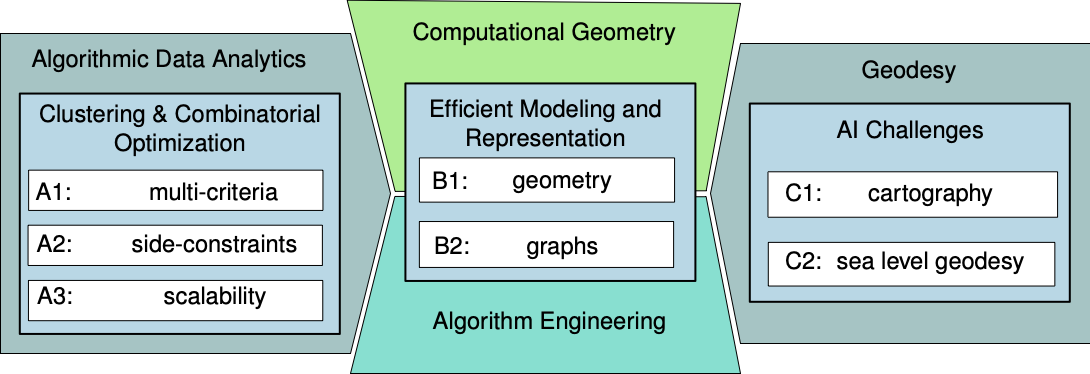Our Team
Our interdisciplinary team consists of members of the University of Bonn, the University of Cologne, and the University of Duesseldorf from the areas of computer science, cartography, and geodesy. We have expertise in, e.g., algorithm engineering, approximation algorithms, cartography, clustering, core sets, Fréchet distance, GIS, graph optimization, interactive maps, multi-objective optimization, smoothed analysis, sea level geodesy, streaming, sublinear algorithms, and triangulations.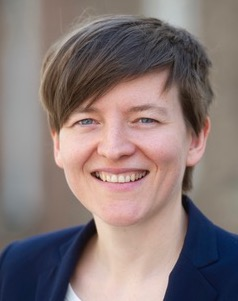Computational Geometry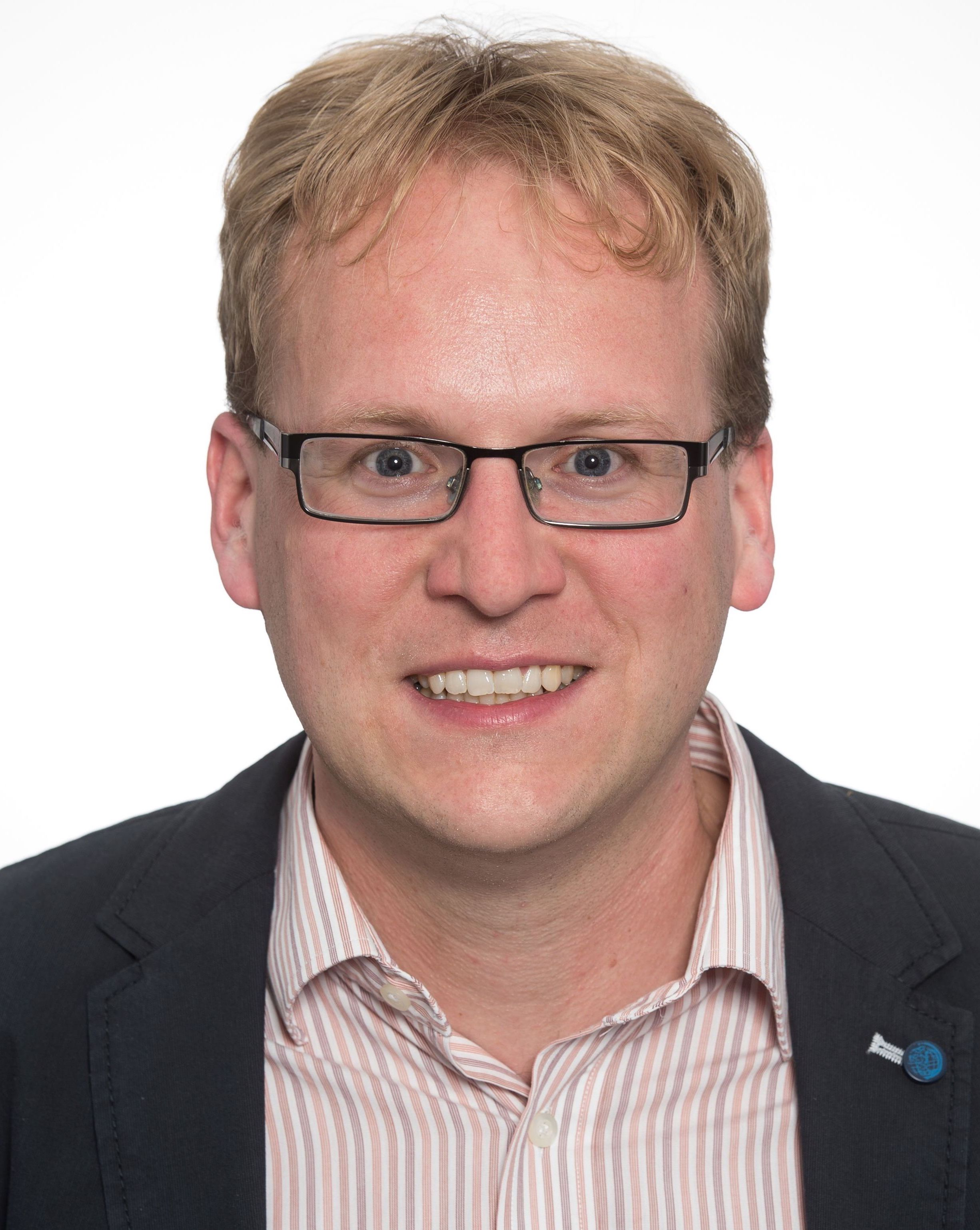Geoinformation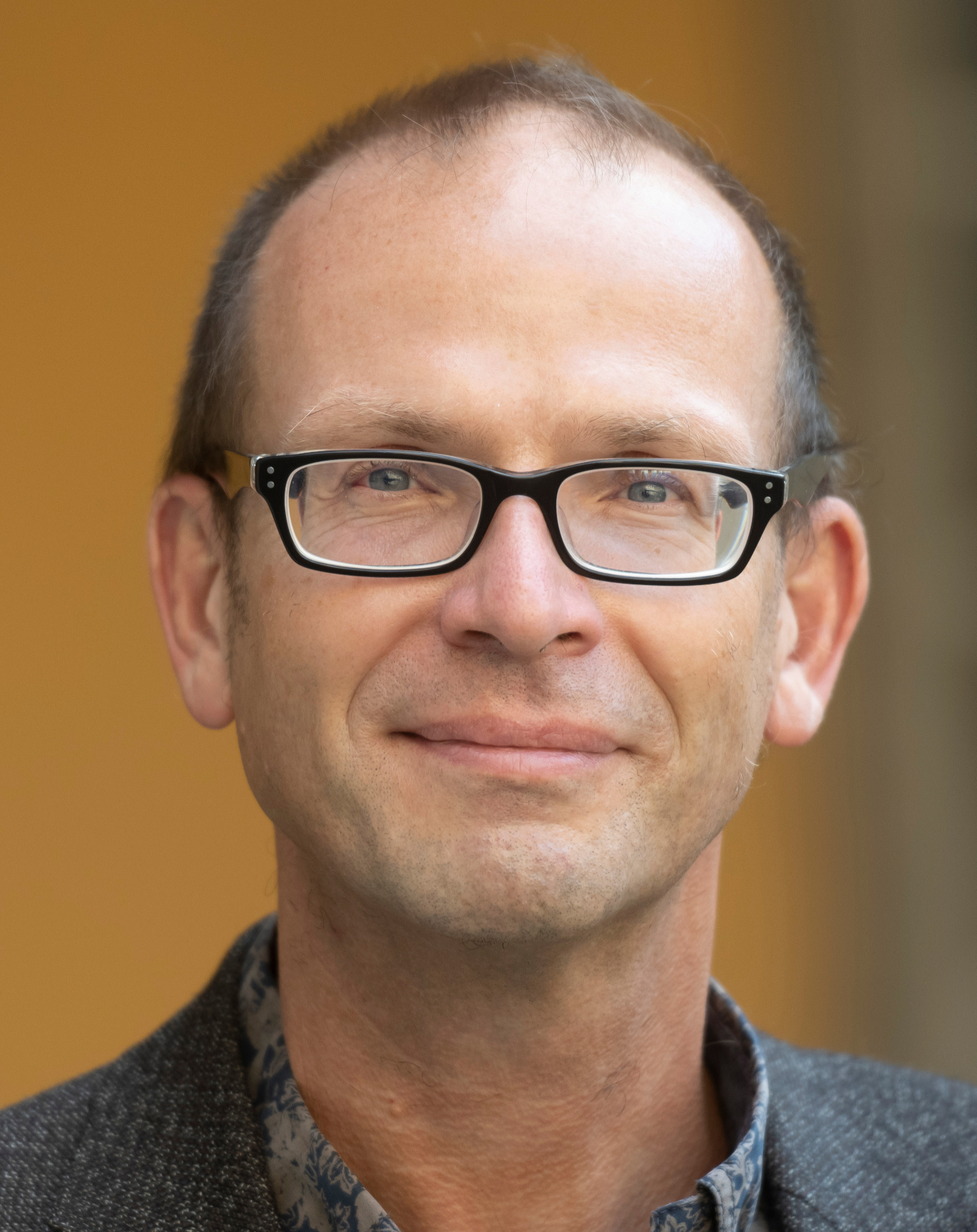Geodesy and Sea LevelComputational AnalyticsTheoretical Computer Science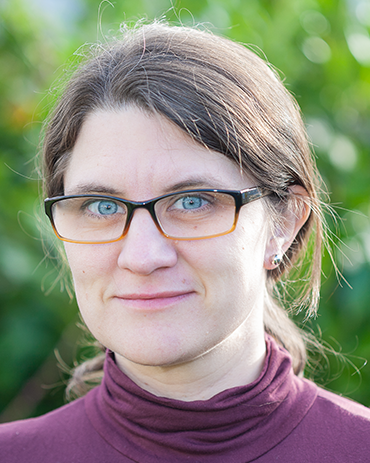Geometric Data Analysis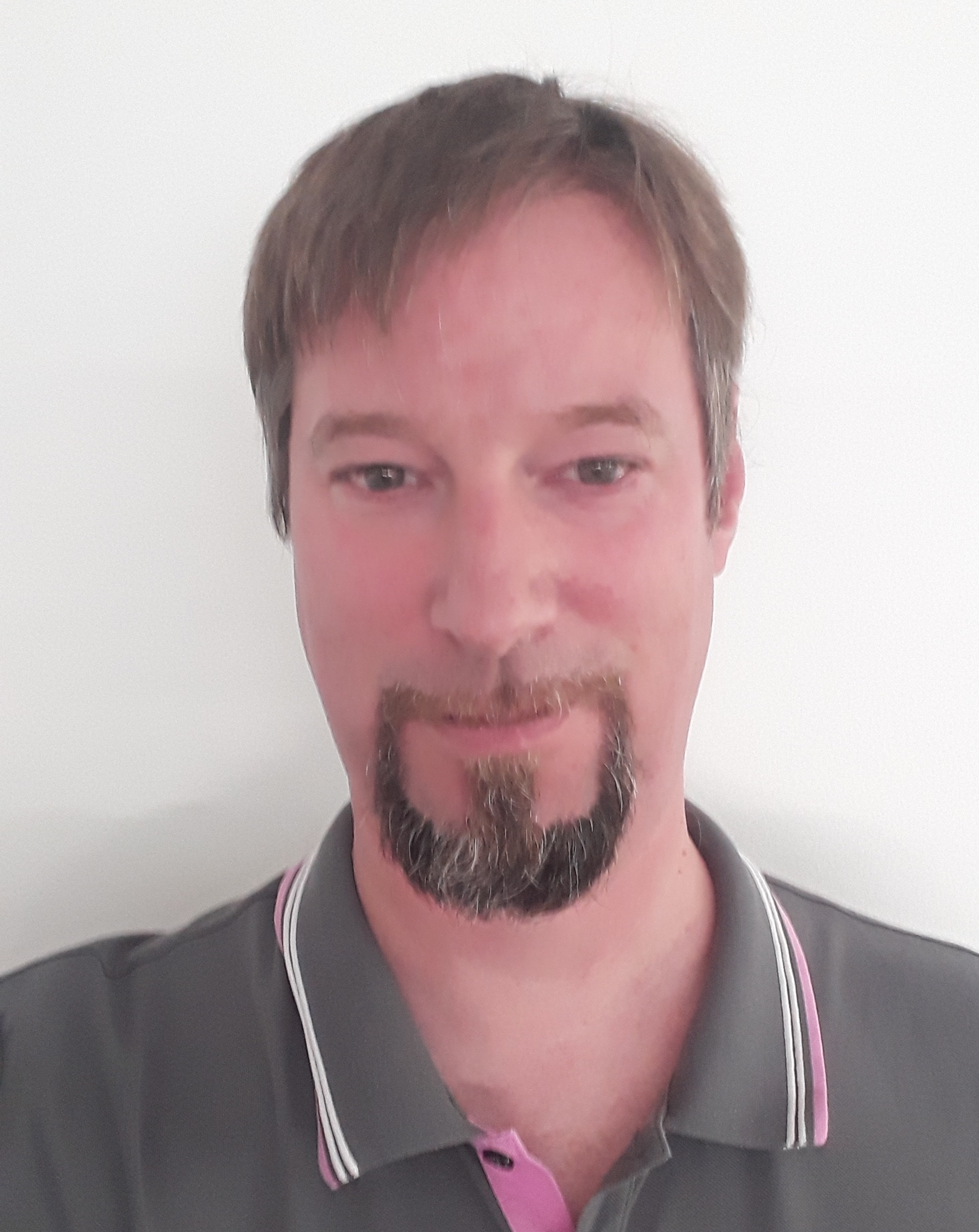Algorithmic Data Analysis